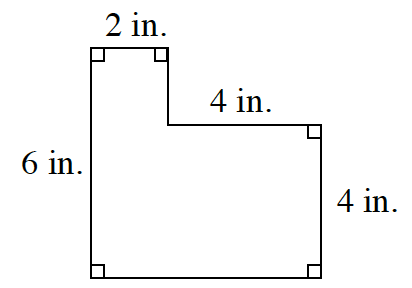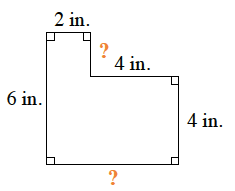### Home > CC2 > Chapter Ch4 > Lesson 4.3.2 > Problem4-99

4-99.

Find the perimeter of the figure below. Homework Help ✎Remember, perimeter is the total length of all the sides of a shape.

What are the missing lengths for the sides of the shape? Try to fill these in and sum up each side length to get the perimeter.

$2+2+4+4+6+6=24$ in.

Make sure you can justify your answer.